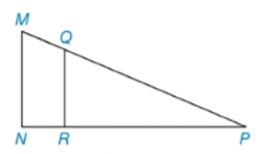Chapter 5.3, Problem 17EElementary Geometry For College St...

7th Edition
Alexander + 2 others
ISBN: 9781337614085

Solutions

Chapter
SectionElementary Geometry For College St...

7th Edition
Alexander + 2 others
ISBN: 9781337614085
Textbook Problem

In Exercises 17 to 24, complete each proof.Given: M N ¯ ⊥ N P ¯ , Q R ¯ ⊥ R P ¯ Prove: Δ M N P ∼ Δ Q R PExercises 17, 18 PROOF Statements Reasons 1. ? 1. ? 2. ∠ s   N and Q R P are right ∠ s 2. ? 3. ? 3. All right ∠ s are ≅ 4. ∠ P ≅ ∠ P 4. ? 5. ? 5.?

To determine

To complete:

The proof by using given information.

Explanation

Given:

Given: MN¯NP¯,QR¯RP¯

Prove: ΔMNPΔQRP

 PROOF Statements Reasons 1. ? 1. ? 2. ∠s N and QRP are right ∠s 2. ? 3. ? 3. All right ∠s are ≅ 4. ∠P≅∠P 4. ? 5. ? 5.?

Definition:

AA:

If two angles of one triangle are congruent to two angles of another triangle, then the triangles are similar.

Description:

Given that MN¯NP¯,QR¯RP¯.

To Prove: ΔMNPΔQRP

Since two lines MN and NP are perpendicular, they form a right triangle. So that sN and QRP are right s.

It is known that all right angles are congruent. Therefore, NQRP.

The two triangles are MNP and QRP. Both have the common point P

Still sussing out bartleby?

Check out a sample textbook solution.

See a sample solution

The Solution to Your Study Problems

Bartleby provides explanations to thousands of textbook problems written by our experts, many with advanced degrees!

Get Started

Find more solutions based on key concepts